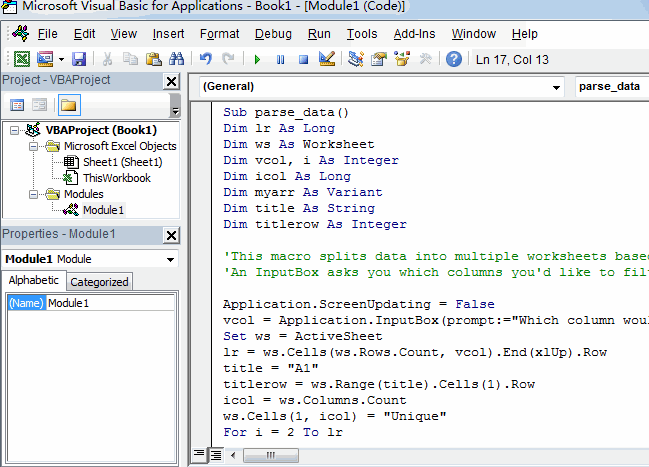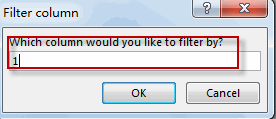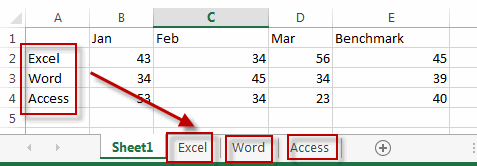Split Data into Multiple Worksheets Based on Column

This post will explain that how to split one worksheet into multiple worksheets based on column value in excel. How do I split a spreadsheet into multiple files based on a column in Excel. How to use VBA macro to split data into different files based on selected column in Excel.

For examples, assuming that you have a file of sales data for all products. And you want to split the data into multiple worksheets based on the product name column. You can refer to the below tutorial to achieve the result.

Split Data into Multiple Worksheets Based on Column value

The best and simple way is to write an Excel VBA macro to split a worksheet into multiple worksheets based on a specified column. And we can use an open source VBA macro from GitHub.

1# click on “Visual Basic” command under DEVELOPER Tab.2# then the “Visual Basic Editor” window will appear.

3# click “Insert” ->”Module” to create a new module.4# paste the below VBA code into the code window. Then clicking “Save” button.```Sub parse_data()
Dim lr As Long
Dim ws As Worksheet
Dim vcol, i As Integer
Dim icol As Long
Dim myarr As Variant
Dim title As String
Dim titlerow As Integer

'This macro splits data into multiple worksheets based on the variables on a column found in Excel.
'An InputBox asks you which columns you'd like to filter by, and it just creates these worksheets.

Application.ScreenUpdating = False
vcol = Application.InputBox(prompt:="Which column would you like to filter by?", title:="Filter column", Default:="3", Type:=1)
Set ws = ActiveSheet
lr = ws.Cells(ws.Rows.Count, vcol).End(xlUp).Row
title = "A1"
titlerow = ws.Range(title).Cells(1).Row
icol = ws.Columns.Count
ws.Cells(1, icol) = "Unique"
For i = 2 To lr
On Error Resume Next
If ws.Cells(i, vcol) <> "" And Application.WorksheetFunction.Match(ws.Cells(i, vcol), ws.Columns(icol), 0) = 0 Then
ws.Cells(ws.Rows.Count, icol).End(xlUp).Offset(1) = ws.Cells(i, vcol)
End If
Next

myarr = Application.WorksheetFunction.Transpose(ws.Columns(icol).SpecialCells(xlCellTypeConstants))
ws.Columns(icol).Clear

For i = 2 To UBound(myarr)
ws.Range(title).AutoFilter field:=vcol, Criteria1:=myarr(i) & ""
If Not Evaluate("=ISREF('" & myarr(i) & "'!A1)") Then
Else
Sheets(myarr(i) & "").Move after:=Worksheets(Worksheets.Count)
End If
ws.Range("A" & titlerow & ":A" & lr).EntireRow.Copy Sheets(myarr(i) & "").Range("A1")
'Sheets(myarr(i) & "").Columns.AutoFit
Next

ws.AutoFilterMode = False
ws.Activate
Application.ScreenUpdating = True
End Sub```

5# back to the current worksheet, then run the above excel macro. Click Run button.6# type the column number that you want to split.7# Let’s see the last result.You might also like:

•Josh says: